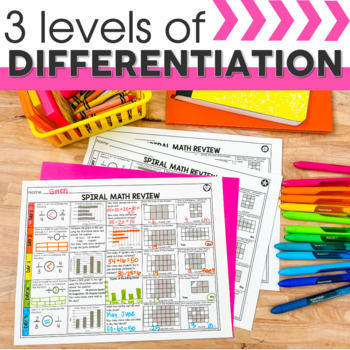# 3rd Grade Math Spiral Review | 3rd Grade Homework | Distance LearningSubject
Resource Type
File Type

Zip

(100 MB|247 pages)
Standards
4 Products in this Bundle
4 products
1. This 3rd grade resource is perfect for morning work, homework, daily math review, and independent math centers.3rd grade spiral math review for first quarter includes 10 weeks of spiral math sheets with 3 differentiated levels and answer keys.What's Included in 1st Quarter Spiral Math Review Sheets:
2. This 3rd grade resource is perfect for morning work, homework, daily math review, and independent math centers.3rd grade spiral math review for second quarter includes 10 weeks of spiral math sheets with 3 differentiated levels and answer keys. What's Included in 2nd Quarter Spiral Math Review Shee
3. This 3rd grade resource is perfect for morning work, homework, daily math review, and independent math centers.3rd grade spiral math review for third quarter includes 10 weeks of spiral math sheets with 3 differentiated levels and answer keys. What's Included in 3rd Quarter Spiral Math Review Sheet
4. This 3rd grade resource is perfect for morning work, homework, daily math review, and independent math centers.3rd grade spiral math review for fourth quarter includes 10 weeks of spiral math sheets with 3 differentiated levels and answer keys. What's Included in 4th Quarter Spiral Math Review Shee
Bonus
1 file
Differentiation Symbol Posters
• Bundle Description
• Standards

This 3rd grade resource WITH 3 LEVELS OF DIFFERENTIATION is perfect for morning work, homework, daily math review, and independent math centers.

3rd grade spiral math review for the ENTIRE YEAR includes 40 weeks of spiral math sheets with answer keys.

What's Included in the Spiral Math Review Sheets BUNDLE:

►At A Glance Sheet

►40 Weeks of Spiral Math Review Sheets

►3 Levels of Differentiation: BELOW, AT, and ABOVE GRADE LEVEL

►Common Core aligned
►Optional blank template to copy to back for additional work space

►Detailed explanation of how to use the resource

Skills included in the Spiral Math Review Sheets BUNDLE:

FIRST QUARTER:

►Round to Nearest 10 and 100

►Two Step Word Problems (Addition & Subtraction)

►Multiples of 10

►Multiplication Strategies

►Mixed Review of 1st Quarter Concepts

SECOND QUARTER:

►Multiplication Strategies

►Multiplication & Division Strategies

►Multiplication & Division within 100 Word Problems

►Determine Unknown Number in Multiplication & Division

►Apply Strategies to Multiply & Divide

►Understand Division as an Unknown Factor Problem

►Fluently Divide within 100

►Two Step Word Problems (Multiplication & Division)

►Data & Graphs (Picture Graphs)

►Mixed Review of 2nd Quarter Concepts

THIRD QUARTER:

►Time to Nearest Minute & Elapsed Time

►Classifying Shapes

►Partition Shapes & Name Fractions

►Fractions on a Number Line

►Fraction Word Problems Using a Number Line

►Identifying Equivalent Fractions

►Whole Numbers as Fractions

►Comparing Fractions

►Data & Graphs (Bar Graphs)

►Mixed Review of 3rd Quarter Concepts

FOURTH QUARTER:

►Area Counting Square Units

►Area Using Multiplication

►Area of Two Figures

►Perimeter Word Problems

►Measure & Estimate Liquid Volumes
►Measurement of Length & Line Plots

►Mixed Review of ALL 3rd Grade Concepts (4 weeks)

▼▼▼▼▼▼▼▼▼▼▼▼▼▼▼▼▼▼▼▼▼▼▼▼▼▼▼▼▼▼▼▼▼▼▼▼▼▼▼▼▼▼▼▼

If you would like to get updates on NEW and CURRENT resources...

▼▼▼▼▼▼▼▼▼▼▼▼▼▼▼▼▼▼▼▼▼▼▼▼▼▼▼▼▼▼▼▼▼▼▼▼▼▼▼▼▼▼▼▼

Permission to copy for single classroom use only.

Electronic distribution limited to single classroom use only.

Not for public display.

Use tiling to show in a concrete case that the area of a rectangle with whole-number side lengths 𝘢 and 𝘣 + 𝘤 is the sum of 𝘢 × 𝘣 and 𝘢 × 𝘤. Use area models to represent the distributive property in mathematical reasoning.
Partition shapes into parts with equal areas. Express the area of each part as a unit fraction of the whole. For example, partition a shape into 4 parts with equal area, and describe the area of each part as 1/4 of the area of the shape.
Understand that shapes in different categories (e.g., rhombuses, rectangles, and others) may share attributes (e.g., having four sides), and that the shared attributes can define a larger category (e.g., quadrilaterals). Recognize rhombuses, rectangles, and squares as examples of quadrilaterals, and draw examples of quadrilaterals that do not belong to any of these subcategories.
Compare two fractions with the same numerator or the same denominator by reasoning about their size. Recognize that comparisons are valid only when the two fractions refer to the same whole. Record the results of comparisons with the symbols >, =, or <, and justify the conclusions, e.g., by using a visual fraction model.
Express whole numbers as fractions, and recognize fractions that are equivalent to whole numbers. Examples: Express 3 in the form 3 = 3/1; recognize that 6/1 = 6; locate 4/4 and 1 at the same point of a number line diagram.
Total Pages
247 pages
Included
Teaching Duration
1 Year
Report this Resource to TpT
Reported resources will be reviewed by our team. Report this resource to let us know if this resource violates TpT’s content guidelines.